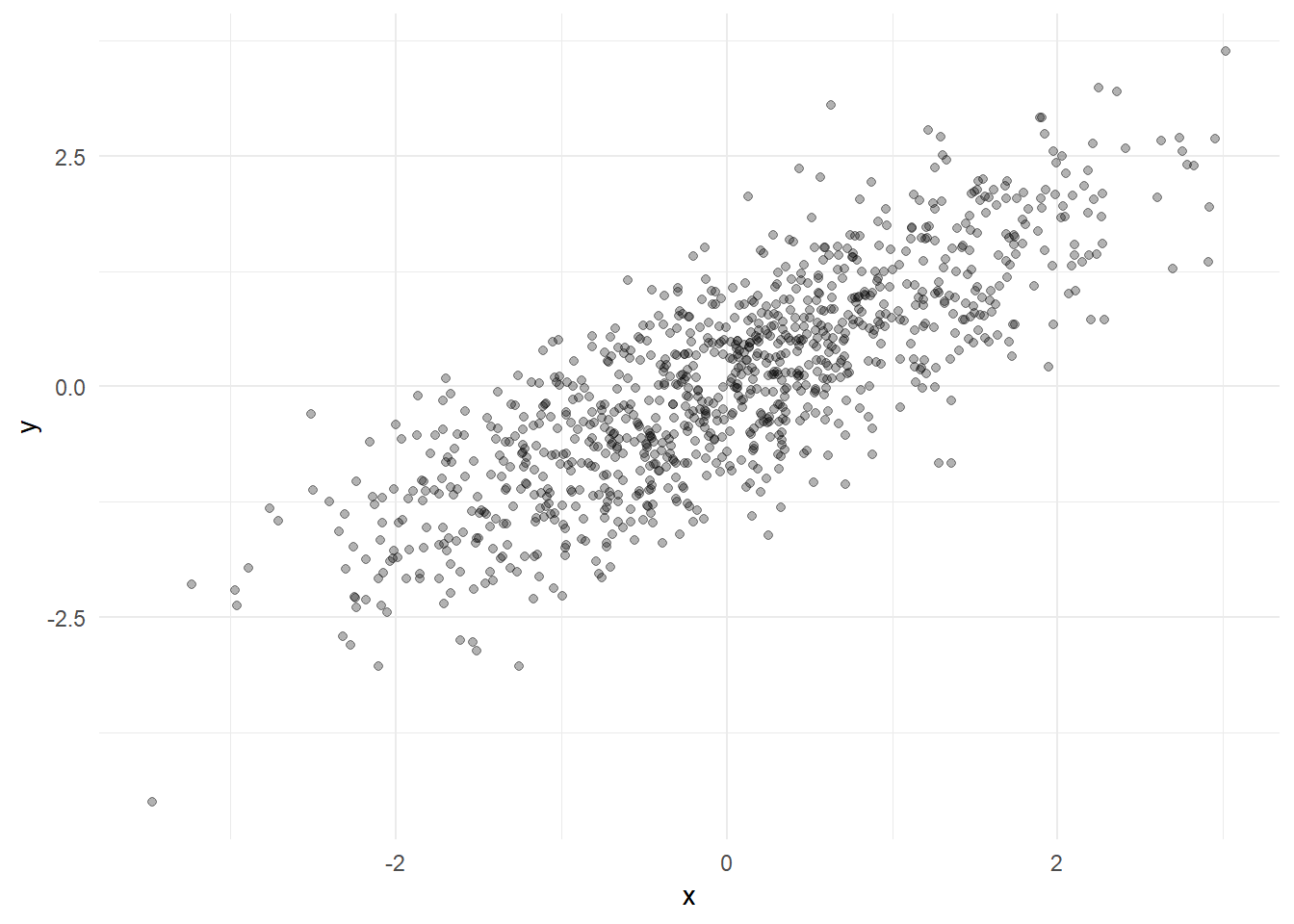This document contains 3-D graphs. You can rotate by dragging and you can zoom with a mouse wheel or two-finger scrolling.

Suppose that we want to know about the effect of `x` on `y` and that we observe that `x` and `y` are correlated. It could be that `x` causes `y`, that `y` causes `x`, or that there is some unknown factor that explains the relationship. For example, there could be a variable (call it `z`) that causes both `x` and `y`. The idea of multiple regression or “controls” in a regression is that if we can observe `z`, then we can see if `x` and `y` are still correlated for subsets of data with equal values of `z`. If `x` and `y` are still related even when `z` does not change, then the relationship between them is not just a result of `z`.

We will create 1000 observations with values of three variables for each observation. By construction, `z` is a standard normal variable, and `x` and `y` are each caused by `z` but do not cause each other.

``````n = 1000
z = rnorm(n)
x = z + .5*rnorm(n)
y = z + .5*rnorm(n)``````
As seen in Figure 1, because `x` and `y` are each related to `z`, they are correlated even though there is no causal relationship between them.Figure 1: x and y are correlated We start exploring controlling for `z` by plotting the data in three dimensions, as in Figure 2. It is hard to tell by just looking at the graph, by `x` and `y` are only related because each increases as `z` increases. You can rotate the plot by dragging, and you can zoom in and out with a mouse wheel, with two finger scrolling, and possibly some other ways. Let’s select a subsample of the data with values of `z` between -0.1 and 0.1 (this is an approximation to holding `z` contant at 0) and plot those points in a different color: